Miscellaneous

Chapter 15 Class 11 Statistics
Serial order wise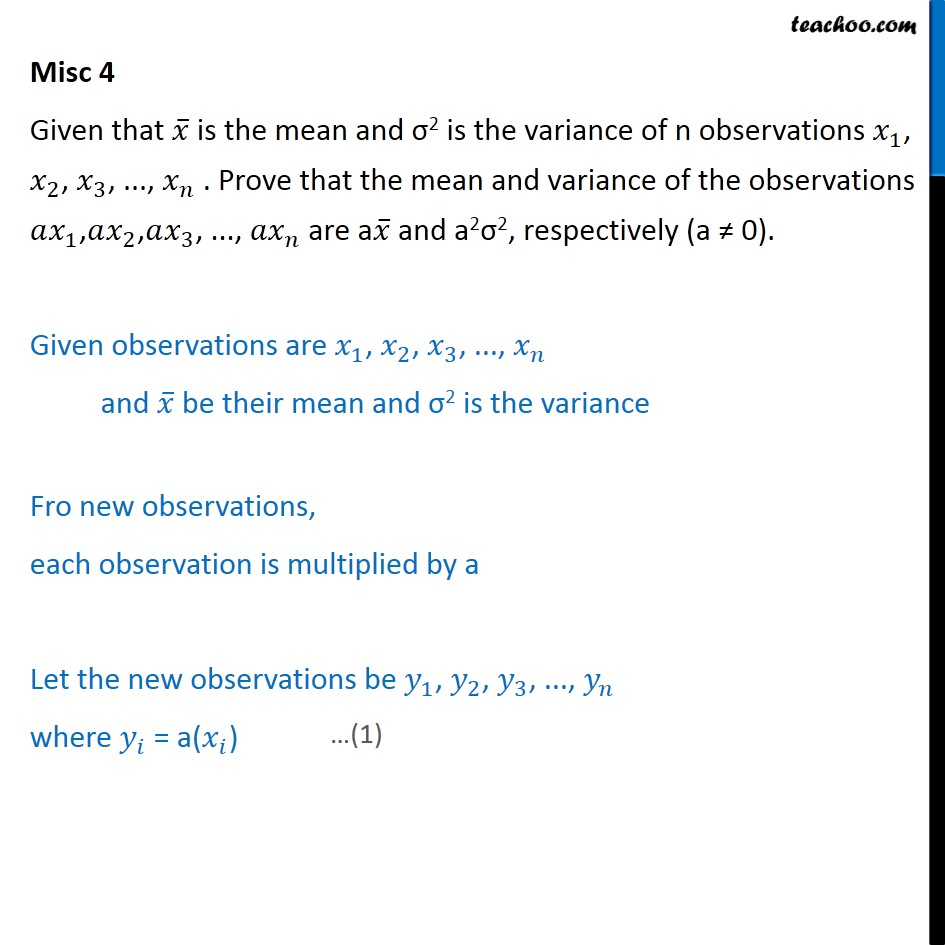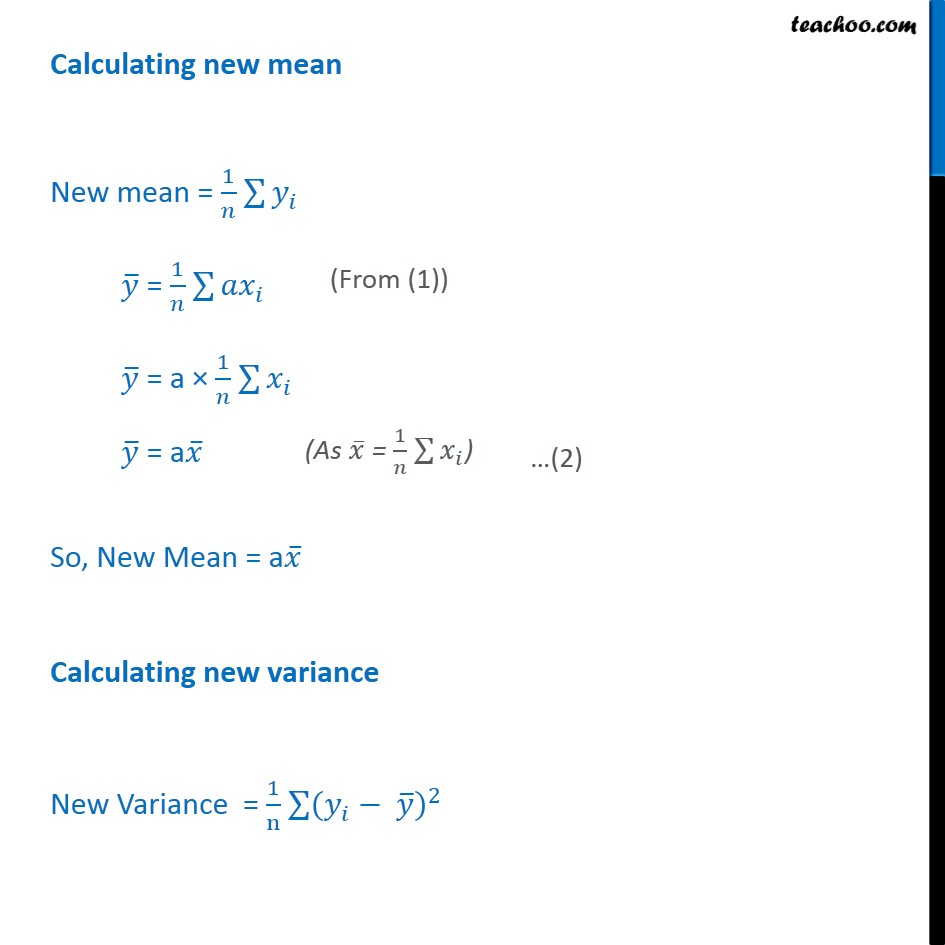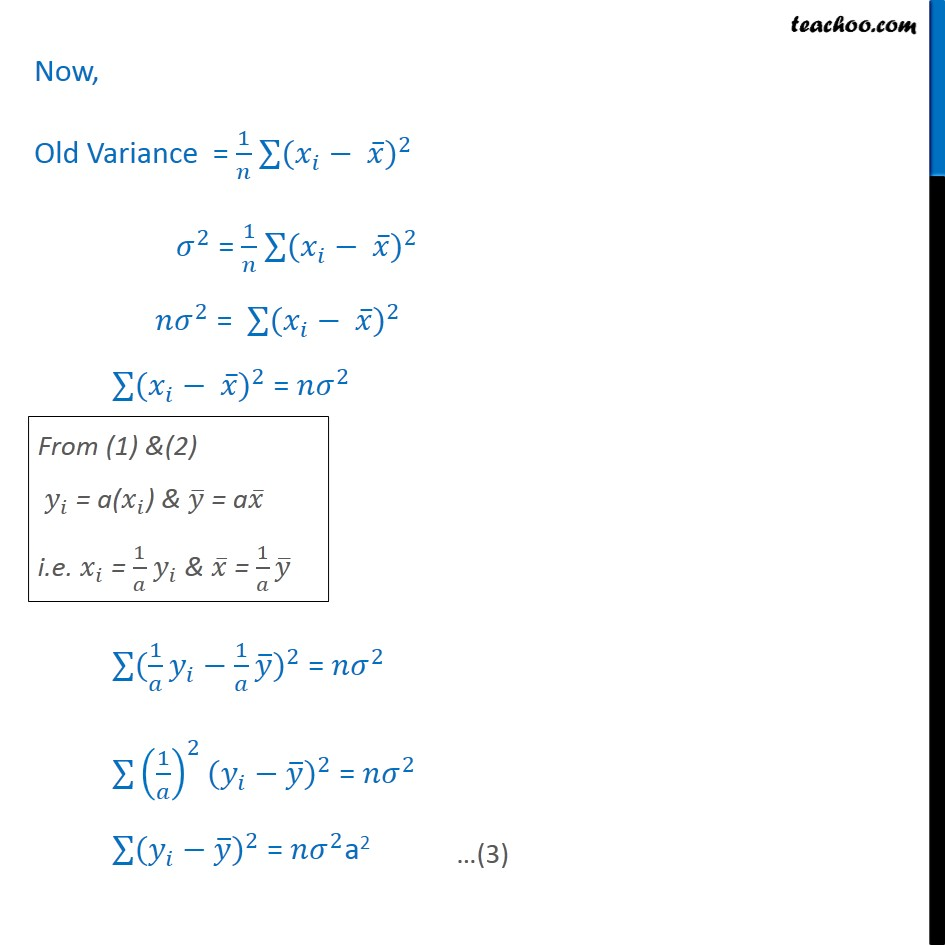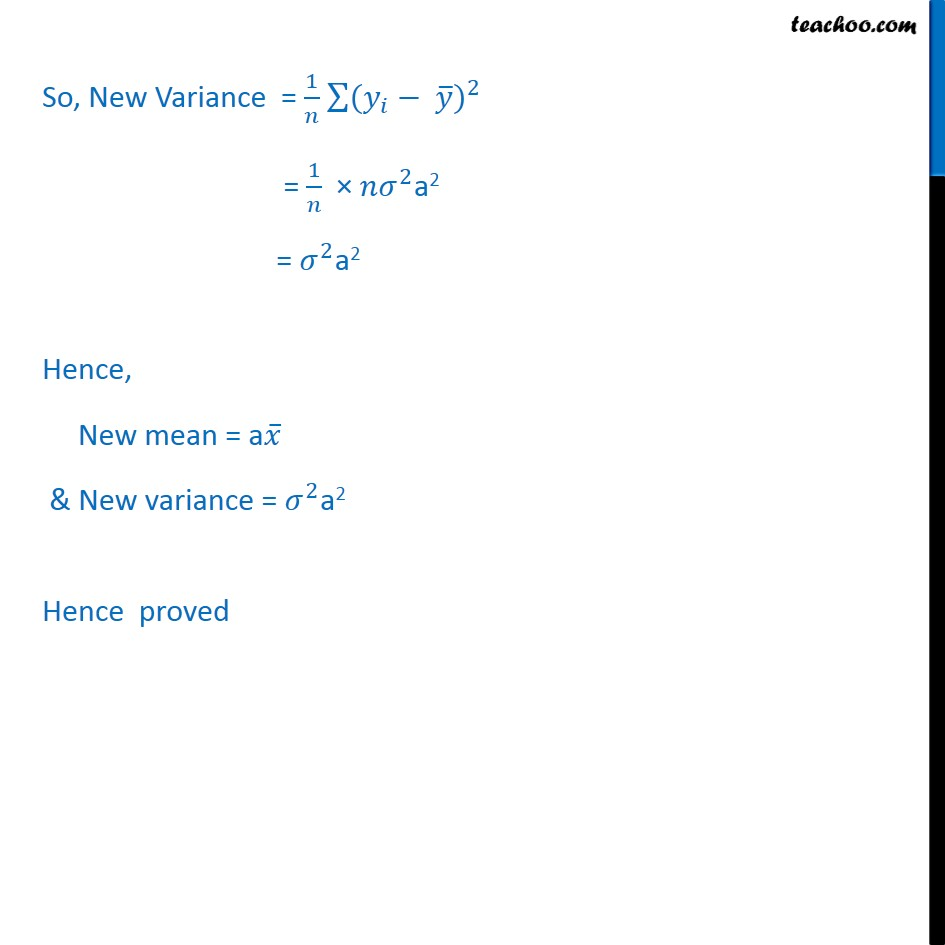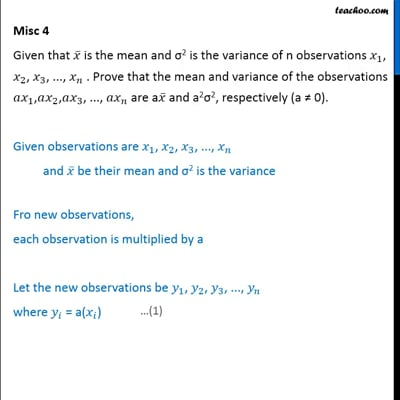This video is only available for Teachoo black users

Maths Crash Course - Live lectures + all videos + Real time Doubt solving!

### Transcript

Misc 4 Given that is the mean and 2 is the variance of n observations 1 , 2 , 3 , ..., . Prove that the mean and variance of the observations 1 , 2 , 3 , ..., are a and a2 2, respectively (a 0). Given observations are 1 , 2 , 3 , ..., and be their mean and 2 is the variance Fro new observations, each observation is multiplied by a Let the new observations be 1 , 2 , 3 , ..., where = a( ) Calculating new mean New mean = 1 = 1 = a 1 = a So, New Mean = a Calculating new variance New Variance = 1 n ( ) 2 Now, Old Variance = 1 ( ) 2 2 = 1 ( ) 2 2 = ( ) 2 ( ) 2 = 2 ( 1 1 ) 2 = 2 1 2 ( ) 2 = 2 ( ) 2 = 2 a2 So, New Variance = 1 ( ) 2 = 1 2 a2 = 2 a2 Hence, New mean = a & New variance = 2 a2 Hence proved